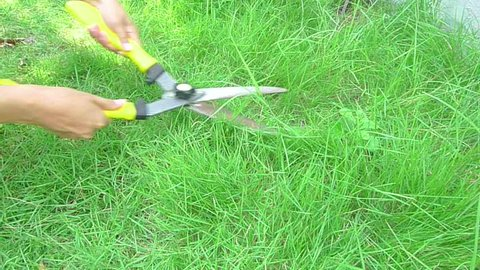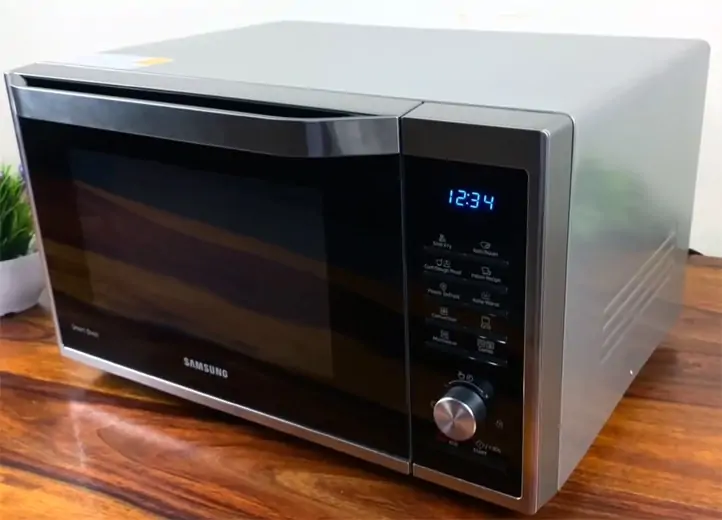# Power Study Guide

INTRODUCTION

Would you rather use a lawnmower or a pair of scissors to cut your lawn grass? A lawnmower would most likely be the answer. What is the reason for this? The lawnmower will complete the operation in a fraction of the time and thus offer you more power. But, exactly, what is power? Let’s take a closer look at this.Source

## WHAT IS POWER?

• In physics, the definition of power is the rate at which work is completed.
• It is the quantity of energy used in a certain amount of time.
• Because energy transfer can be utilized to perform work, power also refers to the rate at which that work is completed.
• When carrying a bottle up a flight of stairs, whether the guy carrying it walks or runs, the work is done in the same amount of time, but more power is spent during the running because the job is done in a smaller duration of time.

## HOW DO WE MEASURE POWER?

• The watt is the standard unit for measuring power, with the symbol  W( SI unit of power).
• James Watt, a Scottish inventor and industrialist, is honored with the unit’s name.
• In your daily life, you’ve most likely come across the watt.
• Watts are commonly used to describe the energy output of electrical devices such as light bulbs and microwaves.

## FORMULA OF POWER

Power is a metric that is measured over a period of time,  which has something to do with how quickly work is completed. The formula for calculating power is given below.

P=W/T,

Where W is the work and T is the time, and P is the power.

## TYPES OF POWER

There are two sorts of power: instantaneous and average.

• Instantaneous power is the power at a given time.
• The definition of average power states that it is the average of all the instantaneous power.

## AN EXAMPLE OF POWERSource

• In just 7 seconds, a microwave oven can produce 7,000 joules of energy. What is its power?

Using the formula of power, which is P= W/T, we can get the power of the microwave.

Power = work / time = 7000J / 7s = 1000 watt .

## SUMMARY

•  Power is a measure of the rate of doing work.
• The SI unit of power is the watt ( W).
• The formula of power is work/time.
• Power can be calculated as Instantaneous or average.

## FAQs

Q. What is power?

Power is the rate at which work is completed.

Q. How do you calculate energy from power?

Energy is the product of power with the length of the time it was consumed.

Q. How are work energy and power related to each other?

Power is the ratio of work energy and the length of time it was consumed.

We hope you enjoyed studying this lesson and learned something cool about Power! Join our Discord community to get any questions you may have answered and to engage with other students just like you! Don’t forget to download our App to experience our fun VR classrooms – we promise, it makes studying much more fun!😎

## REFERENCE

1. Power: https://www.ck12.org/physics/power/lesson/Power-MS-PS/?referrer=concept_details. Accessed 11th April 2022.
2. Power: https://en.wikipedia.org/wiki/Power.Accessed 11th April 2022.
]]>